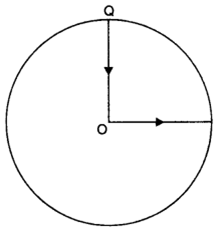• +91 9971497814
• info@interviewmaterial.com

# Chapter 4- Motion in a plane Interview Questions Answers

### Related Subjects

Question 1 : State, for each of the following physical quantities, if it is a scalar or a vector: volume, mass, speed, acceleration, density, number of moles, velocity, angular frequency, displacement, angular velocity.

Scalars: Volume, mass, speed, density, number of moles, angular frequency.
Vectors: Acceleration, velocity, displacement, angular velocity.

Question 2 :
Pick out the two scalar quantities in the following list:
force, angular momentum, work, current, linear momentum, electric field, average velocity, magnetic moment, relative velocity.

Pick out the two scalar quantities in the following list:
force, angular momentum, work, current, linear momentum, electric field, average velocity, magnetic moment, relative velocity.

Question 3 :
Pick out the only vector quantity in the following list:
Temperature, pressure, impulse, time, power, total path length, energy, gravitational potential, coefficient of friction, charge.

Question 4 :
State with reasons, whether the following algebraic operations with scalar and vector physical quantities are meaningful:
(a) adding any two scalars, (b) adding a scalar to a vector of the same dimensions, (c) multiplying any vector by any scalar, (d) multiplying any two scalars, (e) adding any two vectors, (f) adding a component of a vector to the same vector.

(a) No, because only the scalars of same dimensions can be added.
(b) No, because a scalar cannot be added to a vector.
(c) Yes, multiplying a vector with a scalar gives the scalar (number) times the vector quantity which makes sense and one gets a bigger vector. For example, when acceleration A is multiplied by mass m, we get a force F = ml
(d) Yes, two scalars multiplied yield a meaningful result, for example multiplication of rise in temperature of water and its mass gives the amount of heat absorbed by that mass of water.
(e) No, because the two vectors of same dimensions can be added.
(f) Yes, because both are vectors of the same dimensions.

Question 5 :
Read each statement below carefully and state with reasons, if it is true or false:
(a) The magnitude of a vector is always a scalar.
(b) Each component of a vector is always a scalar.
(c) The total path length is always equal to the magnitude of the displacement vector of a particle.
(d) The average speed of a particle (defined as total path length divided by the time taken to cover the path) is either greater or equal to the magnitude of average velocity of the particle over the same interval of time.
(e) Three vectors not lying in a plane can never add up to give a null vector.

(a) True, magnitude of the velocity of a body moving in a straight line may be equal to the speed of the body.
(b) False, each component of a vector is always a vector, not scalar.
(c) False, total path length can also be more than the magnitude of displacement vector of a particle.
(d) True, because the total path length is either greater than or equal to the magnitude of the displacement vector.
(e) True, this is because the resultant of two vectors will not lie in the plane of third vector and hence cannot cancel its effect to give null vector.

Question 6 : Establish the following inequalities geometrically or otherwise: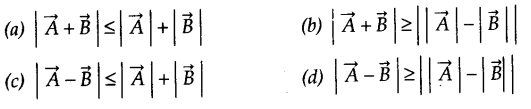When does the equality sign above apply?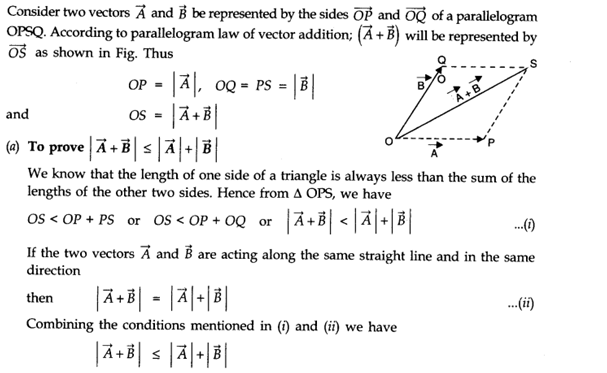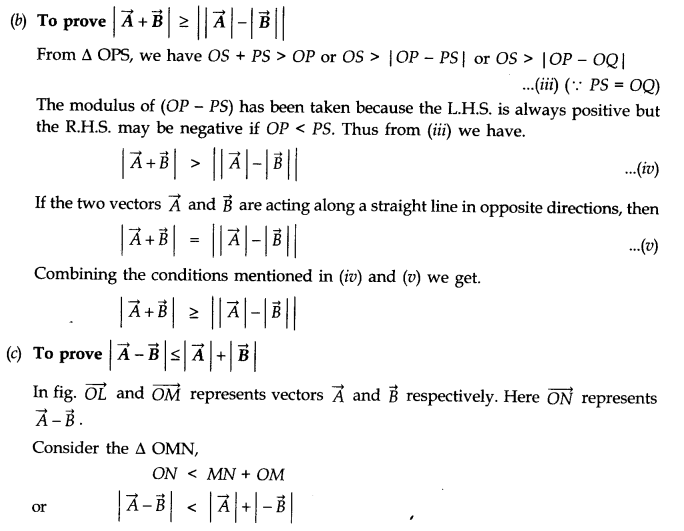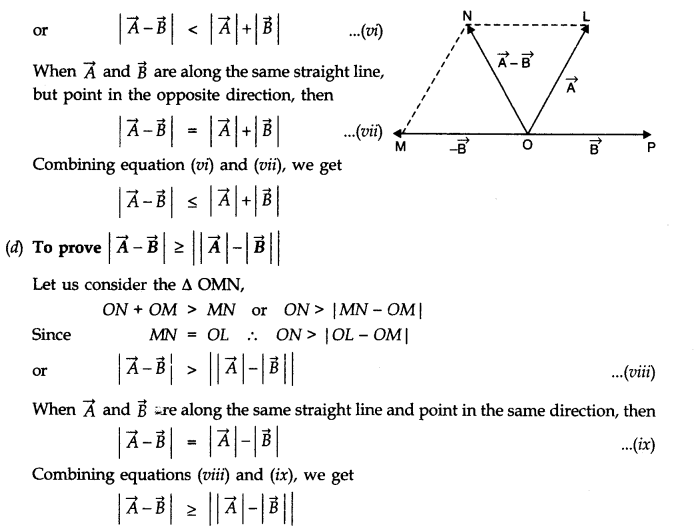Question 7 :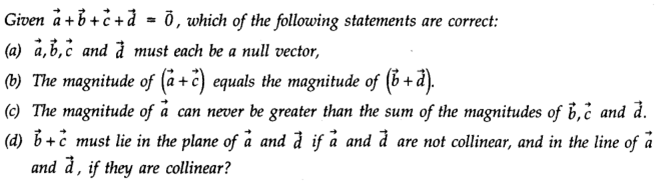Answer 7 :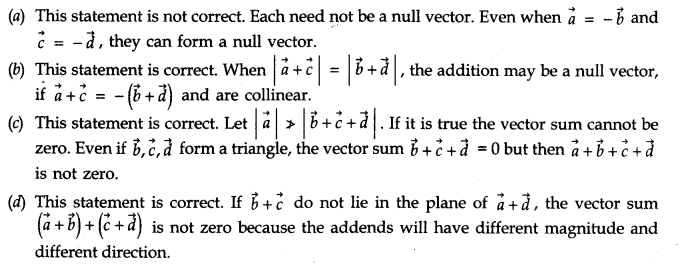Question 8 : Three girls skating on a circular ice ground of radius 200 m start from a point P on the edge of the ground and reach a point Q diametrically opposite to P following different paths as shown in Fig. What is the magnitude of the displacement vector for each? For which girl is this equal to the actual length of path skate?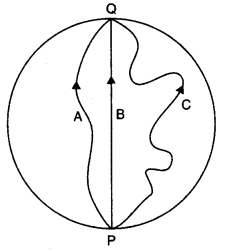Answer 8 :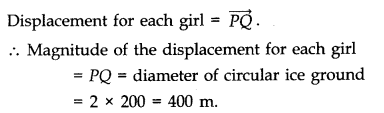Question 9 :
A cyclist starts from the centre O of a circular park of radius 1 km, reaches the edge P of the park, then cycles along the circumference, and returns to the centre along QO as shown in Fig. If the round trip takes 10 min, what is the (a) net displacement,
(b) average velocity, and (c) average speed of the cyclist?